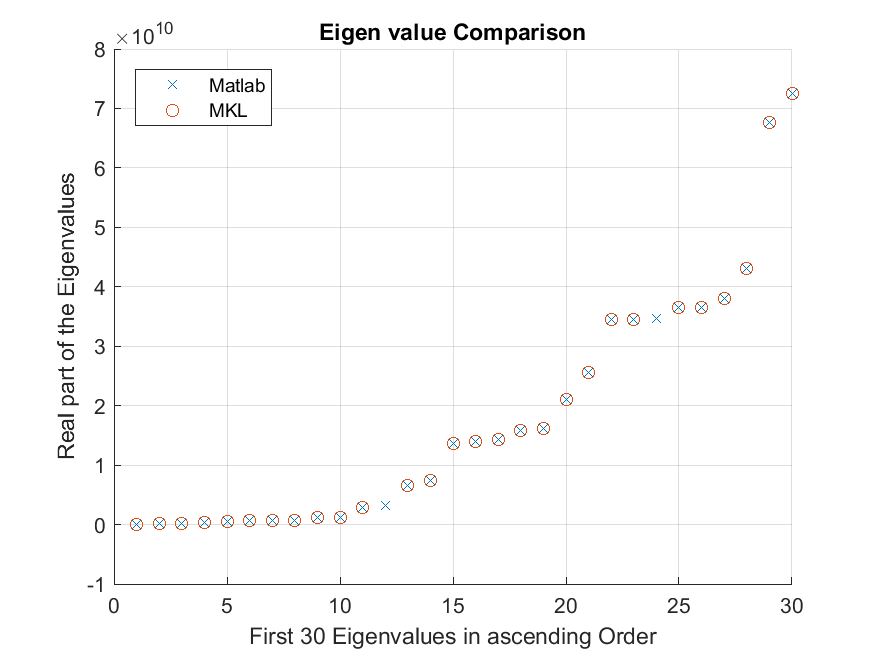Intel® oneAPI Math Kernel Library
Ask questions and share information with other developers who use Intel® Math Kernel Library.
Announcements
6743 Discussions

## Inconsistency finding Eigenvalues for sparse matrices with MKLBeginner
307 Views

Hi,

I am trying to calculate the smallest real eigenvalues of a general problem A*V = B*V*D with sparse matrices using MKL and I have been having four problems when comparing to MATLAB:

1. I am always missing at least one of the smallest eigenvalues in MKL in comparison to MATLAB.

For example2. Using the same matrix most of the eigenvalues I do get are almost Identical between MATLAB and MKL, but which of the eigenvalues I am missing is somewhat random.

Same System, different results3. The smallest eigenvalue tend to be a several orders of magnitudes different between MATLAB and MKL (in some unfrequent cases not only the first)

MATLAB both Graphs: -0.000200

MKL first graph:          380057.918617

MKL second graph:     364053.320270

4. For some sparse matrices describing a very similar system with similar sparcity but different non-zero values I get no eigenvalues on MKL but several hundreds on MATLAB

I attached all data needed to reproduce my results in two Zips docs

Could somebody help me understand what I might be doing wrong or what alternative could I use to solve the problem

Thanks a lot

4 RepliesModerator
307 Views

Andres, is that 32 or 64-bit case? Did you link with the threaded version of mkl? is OpenMP or tbb?Moderator
307 Views

please give us the reproducer shows how to you call mkl's routinesBeginner
307 Views

We compile in x64. We do not want to run in parallel.

Following is the code used to call feast algorithm:

_putenv("OMP_DYNAMIC=FALSE");
_putenv("MKL_DYNAMIC=FALSE");

MKL_INT n = jDof;

// CSR Matrix A
double* a = toArray(env, jVals_A);
MKL_INT* ia = toArray(env, jRows_A);
MKL_INT* ja = toArray(env, jCols_A);

// CSR Matrix B
double* b = toArray(env, jVals_B);
MKL_INT* ib = toArray(env, jRows_B);
MKL_INT* jb = toArray(env, jCols_B);

// search interval & subspace size for solution of eigenvalue problem
double emin = jEmin;
double emax = jEmax;
MKL_INT m0 = jM0;

// solution variables
double* e = new double[m0];
double* x = new double[m0*n];
MKL_INT* m = new MKL_INT;
double* res = new double[m0];

// Eigen solver control parameters
MKL_INT fpm;
MKL_INT loop, info;
double epsout;

/* -------------------------------------------------------------------- */
/* .. Setup eigenvalue solver control parameters. */
/* -------------------------------------------------------------------- */
feastinit(fpm);

fpm = 1;
fpm = 0;              /* Check input matrices */
fpm = 0;              /* Check whether B matrix is positive definite */

info = 0;                 /* Initialize info flag */
const char* uplo = "F";   /* full matrices are provided*/

dfeast_scsrgv(uplo, &n, a, ia, ja, b, ib, jb, fpm, &epsout, &loop, &emin, &emax, &m0, e, x, m, res, &info);

thank you for your interest in trying to help us

Best regards

AndrésBeginner
307 Views

Hello everybody,

do you have updates on my issue? I need a solution urgently.

Best regards

Andrés